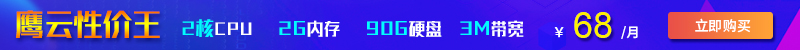这篇文章给大家介绍了“如何搞懂算法的时间复杂度和空间复杂度”的相关知识，讲解详细，步骤过程清晰，对大家进一步学习和理解“如何搞懂算法的时间复杂度和空间复杂度”有一定的帮助，希望大家阅读完这篇文章能有所收获。下面就请大家跟着小编的思路一起来学习一下吧。• 时间维度：是指执行当前算法所消耗的时间，我们通常用「时间复杂度」来描述。

• 空间维度：是指执行当前算法需要占用多少内存空间，我们通常用「空间复杂度」来描述。

## 一、时间复杂度

```for(i=1; i<=n; ++i)
{
j = i;
j++;
}```

• 常数阶O(1)

• 对数阶O(logN)

• 线性阶O(n)

• 线性对数阶O(nlogN)

• 平方阶O(n²)

• 立方阶O(n³)

• K次方阶O(n^k)

• 指数阶(2^n)

• 常数阶O(1)

```int i = 1;
int j = 2;
++i;
j++;
int m = i + j;```

• 线性阶O(n)

```for(i=1; i<=n; ++i)
{
j = i;
j++;
}```

• 对数阶O(logN)

```int i = 1;
while(i<n)
{
i = i * 2;
}```

• 线性对数阶O(nlogN)

```for(m=1; m<n; m++)
{
i = 1;
while(i<n)
{
i = i * 2;
}
}```
• 平方阶O(n²)

```for(x=1; i<=n; x++)
{
for(i=1; i<=n; i++)
{
j = i;
j++;
}
}```

```for(x=1; i<=m; x++)
{
for(i=1; i<=n; i++)
{
j = i;
j++;
}
}```

• 立方阶O(n³)、K次方阶O(n^k)

## 二、空间复杂度

• 空间复杂度 O(1)

```int i = 1;
int j = 2;
++i;
j++;
int m = i + j;```

• 空间复杂度 O(n)

```int[] m = new int[n]
for(i=1; i<=n; ++i)
{
j = i;
j++;
}```

2022-09-01 17:38:53

2022-05-19 17:42:37

2021-11-01 17:56:26• 联系我们
• 24小时售后：4006784567
• 24小时TEL ：0668-2555666
• 售前咨询TEL：400-678-4567
•官方微信官方微信
Copyright  ©  QY  Network  Company  Ltd. All  Rights  Reserved. 2003-2019  群英网络  版权所有   茂名市群英网络有限公司